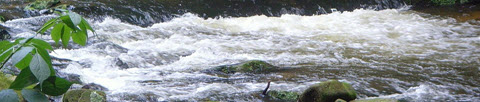# Electricity Water AnalogyDon't mix water and electricity!

But water can help us understand electricity.

They don't behave exactly the same, but similar enough to be helpful when first learning, like this:Let us look at each concept in turn:

### q = Charge

Electric charge (measured in Coulombs) is like quantity of water (liters).A fully charged battery is like a full tank of water.

### I = Current

A flow of electricity is called current because it is similar to a current of water:The electric current (measured in Amps) is like the flow rate of water.

### V = Voltage

Voltage (measured in Volts) is like water pressure. More voltage gives more current! No voltage, no current.

In fact voltage is sometimes called "potential difference": the difference can be thought of like the difference in height that gives the water its pressure, and "potential" because it can be sitting waiting to go.

### R = Resistance

Resistance (measured in Ohms) is like a pipe's resistance to flow. More resistance (such as a clogged or thinner pipe) means less flow (current).

Imagine we put a filter in the pipeline, it will resist the flow of water, and we get less flow. We will need to increase the pressure (voltage) to bring the flow rate back up again.

## Relationship

Voltage (V), Current (I) and Resistance (R) are related by Ohm's Law:

V = IR

It is very useful in electric circuits.

V = IR can be rearranged into I = VR that shows us:More Voltage leads to More Current:More Resistance leads to Less Current:There are other ways that Electricity and Water are similar (but not perfectly so):

## Power

Electrical power is the rate at which electrical energy is transferred. The formula is Power = Current × Voltage:Similar to water where we get more power by increasing pressure and/or flow:## Capacitors

A capacitor holds charge. For water it is a bit like a pipe with a piston and spring:The amount of charge stored in the capacitor depends on its capacitance, just as the amount of water a pipe can hold depends on its volume.

## InductorsAn inductor is like a water wheel with inertia. Just as flowing water causes the wheel to rotate, electric current passing through an inductor generates a magnetic field.

When the flow of water suddenly stops, the water wheel continues to rotate due to its inertia. Similarly, when the flow of electric current is abruptly interrupted, an inductor's magnetic field persists briefly due to its inertia.

A higher inductance means the inductor can store more potential energy in the magnetic field, just like a larger water wheel with more mass can store more rotational energy.

## DiodesA diode is like a one way water valve, only allowing flow in one direction.

## In Conclusion

While there are some similarities between Electricity and Water, they are not the same and they can behave very differently, too.

So while this is helpful when first learning, the similarities do break down as you learn more.